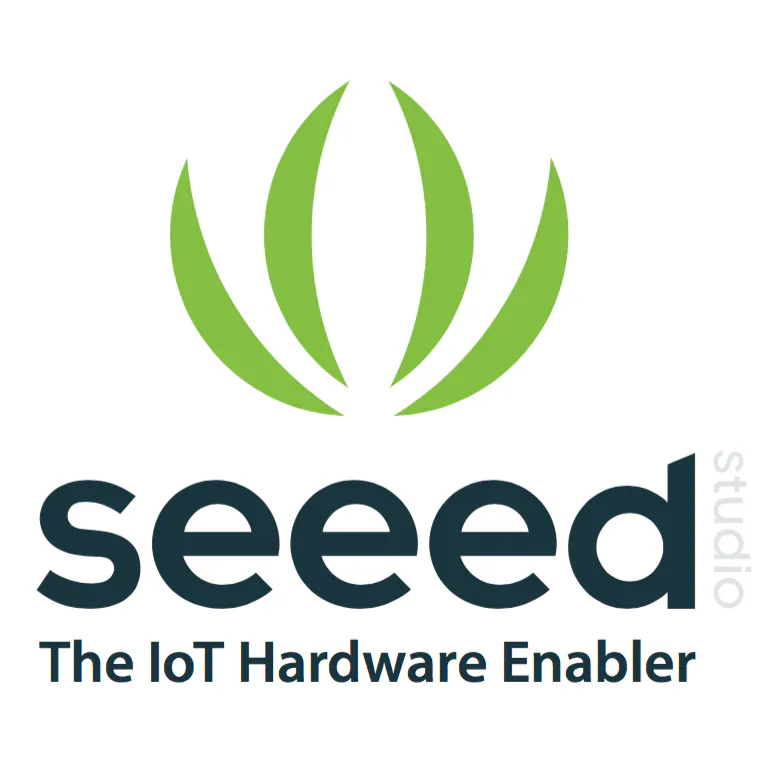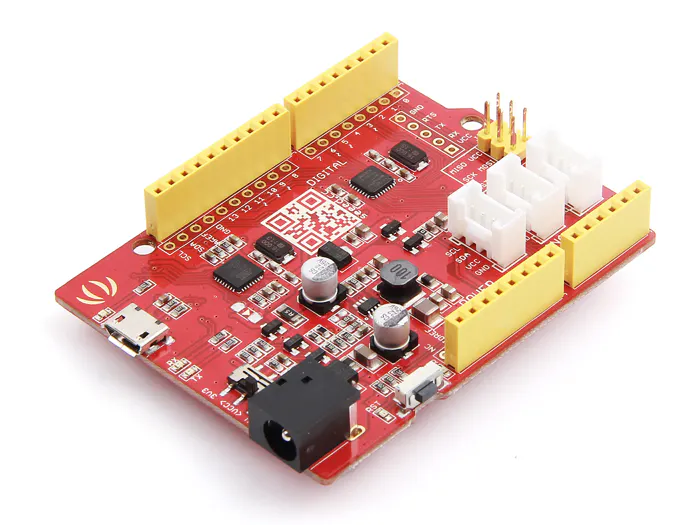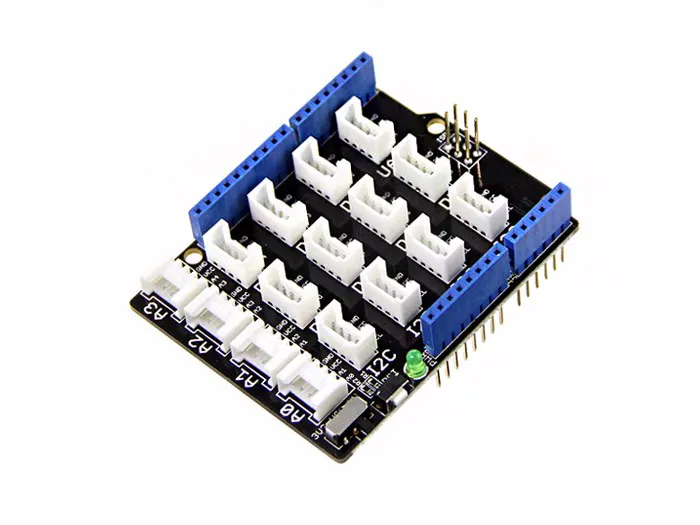Published

# Grove Analog Microphone displaying on Wio Terminal

This project is going to introduce how this Grove-Analog Microphone works via Serial Port and Wio Terminal!

IntermediateFull instructions provided87

## Things used in this project

### Hardware componentsSeeeduino V4.2
×1Seeed Base Shield V2
×1
 Seeed Wio Terminal
×1
 Seeed Grove-Analog Microphone
×1

### Software apps and online servicesArduino IDE

## Code

### Demo Code for Arduino

C/C++
```/*

Reads an analog input pin, maps the result to a range from 0 to 255 and uses
the result to set the pulse width modulation (PWM) of an output pin.
Also prints the results to the Serial Monitor.
*/

// These constants won't change. They're used to give names to the pins used:
const int analogOutPin = 9; // Analog output pin that the LED is attached to

int sensorValue = 0;        // value read from the pot
int outputValue = 0;        // value output to the PWM (analog out)

void setup() {
Serial.begin(9600);      // initialize serial communications at 9600 bps
}

void loop() {
outputValue = map(sensorValue, 0, 1023, 0, 255);       // map it to the range of the analog out
analogWrite(analogOutPin, outputValue);               // change the analog out value

Serial.println(outputValue);    // print the results to the Serial Monitor

// wait 2 milliseconds before the next loop for the analog-to-digital
// converter to settle after the last reading:
delay(2);
}<br>
```

### Demo Code for Wio Terminal

C/C++
```#include "seeed_line_chart.h" //include the library

TFT_eSPI tft;
#define LINE_DIS 0X00
#define STRING_DIS 0X01
#define max_size 30 //maximum size of data
doubles data;       //Initilising a doubles type to store data
int brightness;
TFT_eSprite spr = TFT_eSprite(&tft); // Sprite

const int MIC = A0; //the microphone amplifier output is connected to pin A0
int dB, PdB; //the variable that will hold the value read from the microphone each time
uint8_t mode = LINE_DIS;
void setup()
{
Serial.begin(9600); //sets the baud rate at 9600 so we can check the values the microphone is obtaining on the Serial Monitor
pinMode(A0, INPUT);
pinMode(WIO_KEY_C, INPUT_PULLUP);
pinMode(WIO_BUZZER, OUTPUT);
tft.begin();
spr.createSprite(TFT_HEIGHT, TFT_WIDTH);
spr.setRotation(3);
tft.setRotation(3);
}

void loop() {

mode ++;
if(mode > STRING_DIS ) mode = LINE_DIS;
}
display(mode);
}

void display(uint8_t mode)
{
dB = (adc + 83.2073) / 7.003; //Convert ADC value to dB using Regression values

if(dB > 50)
{
analogWrite(WIO_BUZZER, 128);

}else{
analogWrite(WIO_BUZZER, 0);
}

spr.fillSprite(TFT_WHITE);

if (data.size() == max_size)
{
data.pop(); //this is used to remove the first read variable
}
data.push(dB); //read variables and store in data

//Settings for the line graph title
.align(center)
.valign(vcenter)
.width(tft.width())
.thickness(2);

if (LINE_DIS == mode){
//Settings for the line graph
auto content = line_chart(20, header.height()); //(x,y) where the line graph begins
content
.height(tft.height() - header.height() * 1.5) //actual height of the line chart
.width(tft.width() - content.x() * 2)         //actual width of the line chart
.based_on(0.0)                                //Starting point of y-axis, must be a float
.show_circle(false)                           //drawing a cirle at each point, default is on.
.value(data)                                  //passing through the data to line graph
.color(TFT_RED)                               //Setting the color for the line
.draw();
} else if (STRING_DIS == mode){
for(int8_t line_index = 0;line_index < 5 ; line_index++)
{
spr.drawLine(0, 50 + line_index, tft.width(), 50 + line_index, TFT_GREEN);
}
.thickness(1);
spr.setFreeFont(&FreeSansBoldOblique24pt7b);
if(dB > 50){
spr.setTextColor(TFT_RED);
}else{
spr.setTextColor(TFT_BLUE);
}
spr.drawFloat(dB,2,70,110);
spr.drawString(" dB",80 + 100,110,1);
}
spr.pushSprite(0, 0);
delay(100);
}
```

## Credits

### Seeed

59 projects • 73 followers
Seeed R&D Team